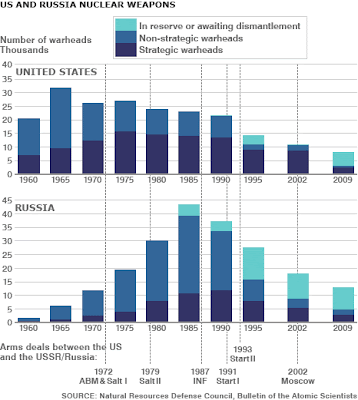## tisdag 19 juli 2011

### Answer to Question by Roy SpencerIn an exchange between Roy Spencer and Marty Hertzberg regarding the proper use of the Stefan-Boltzmann equation in climate modeling, Roy asks the question:
• How does the surface 'know' how opaque the atmosphere is before it 'decides' at what rate it should emit IR?
which can be turned around into:
• How does the Earth's surface 'know' what the temperature of the atmosphere is before it 'decides' at what rate it should emit IR?
Roy asks this question to challenge the view put forward by Marty that the temperature Ta
of the environment (the absorber) of a (blackbody) emitter at temperature Te bigger than Ta, determines the amount of energy E emitted according to Stefan-Boltzmann's law written in the form:
• E = sigma (Te^4 - Ta^4) ~ 4 sigma Te^3 (Te - Ta)
expressing E as a multiple of the difference in temperature Te - Ta. In particular, this means that there is no backradiation of energy from a cold atmosphere to a warmer Earth surface, only the other way.

This is different from a common view adopted by Roy of a two-way emission of photons carrying energy between emitter and absorber, in which Stefan-Boltzman's law would be written
• E = sigma Te^4 - sigma Ta^4
expressing the net energy transfer E as the difference between a two way gross flow of energy
with emitter and absorber both emitting into a background of zero K. In this view, a cold atmosphere would be warming a warmer Earth surface by sigma Ta^4, while the Earth surface
would be transferring sigma Te^4 to the atmosphere.

In the new derivation of Planck's law behind Stefan-Boltzmann presented in Slaying the Sky Dragon, with more details in the upcoming book Mathematical Physics of Blackbody Radiation,
I give support to Marty's standpoint, which is in opposition to Roy's.

Roy's question is how the Earth reads the temperature of the atmosphere and an answer is suggested by my analysis: The emitter and absorber stay in contact by two-way electromagnetic waves described by Maxwell's equations. This contact makes it possible for both emitter and absorber to read the temperature of the other by reading the spectrum of the other.

This is like two superpowers reading the destruction capability of the other without pressing the buttons to exchange of mutual destruction, see picture above.

The basic idea is that the contact allowing temperature reading is established by two-way electromagnetic waves, while the transfer of energy is one-way (from warm to cold).

Mathematically the two versions of Stefan-Boltzmann may look equivalent, but the physics behind the two is different: Roy's is two-way stream of particles carrying energy back and forth.
Marty's and mine is two-way electromagnetic waves carrying information with one-way transfer of energy.

The difference comes out when looking at perturbations of forcing, as perturbations of net one-way flow of energy (small) vs perturbations of gross two-way flow of energy (big). This is the origin of CO2 alarmism: How to make something small into something big.

Does this answer your question Roy?

In support of Marty's and my view, one may add that a precise macroscopic mathematical model for electromagnetic waves is known (Maxwell's equations), while that of photon flight appears to be unknown (unless it is assumed to be a trivial ray model).

#### 2 kommentarer:

1.Claes, this time I agree with you and this is used in most engineering texts books on heat transfer (for example you will see a graph of h(r) as Fig. 5-7 in Perry's Chemical Engineering Handbook). The thing missing is the emissivity factors. The formula is then used in a heat transfer coefficient h(r) which can be added to the convective heat transfer coefficient h(c). The emissivities of combustion products at various temperatures have been extensively studied.
Those people who just use the black body Stefan-Boltzman relation have no understanding or experience with heat transfer.
keep strong
Cementafriend

2.I agree with Cementafriend. Also if you look at the extreme example of Ta = Te then W(E) is zero. Not one iota of energy is passing from anyone to anyone. Now if that is true if absorber is same temperature as emitter then how can absorber added energy to emitter when at a lower temperature. It adds nothing at same temperture.